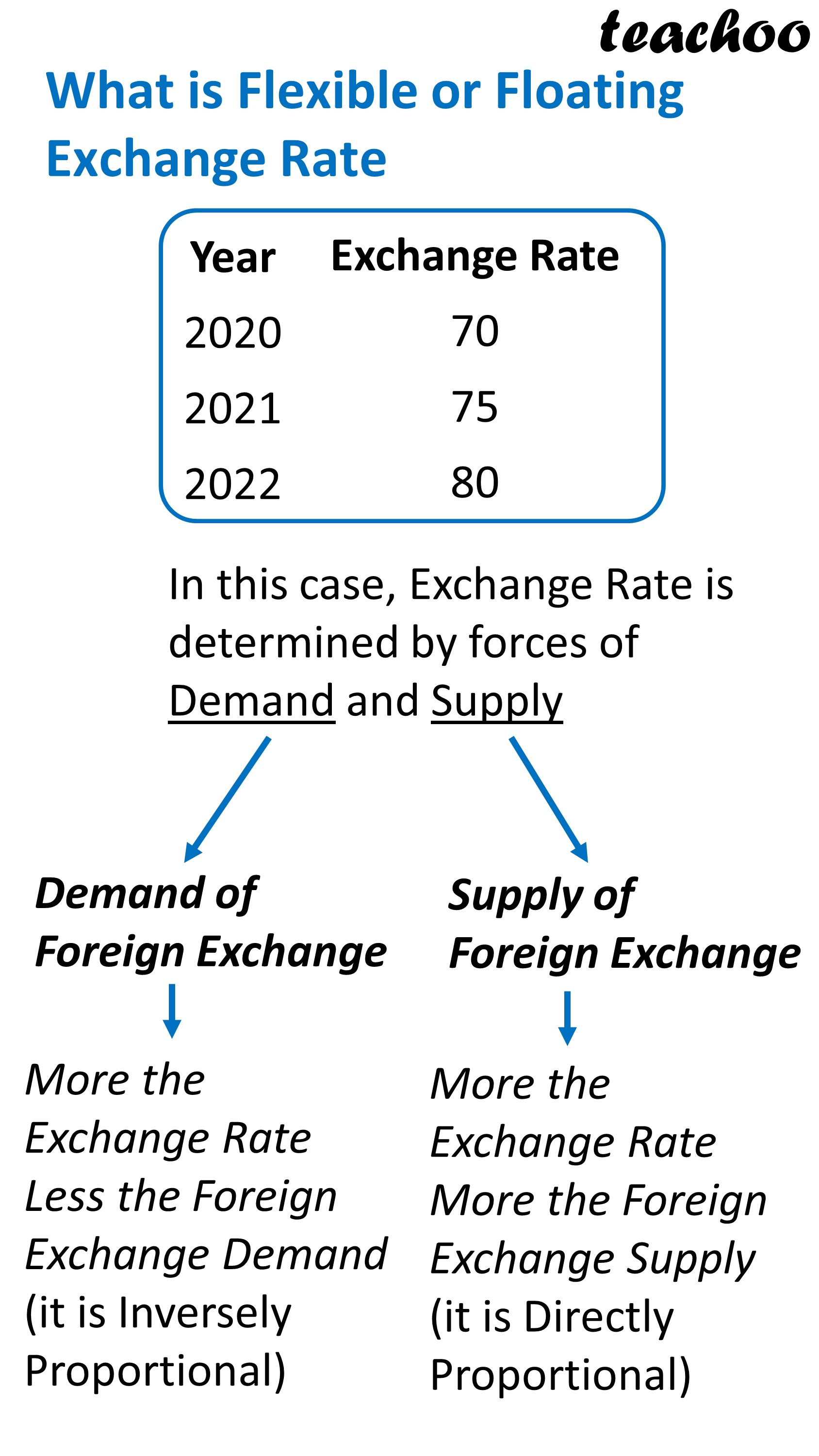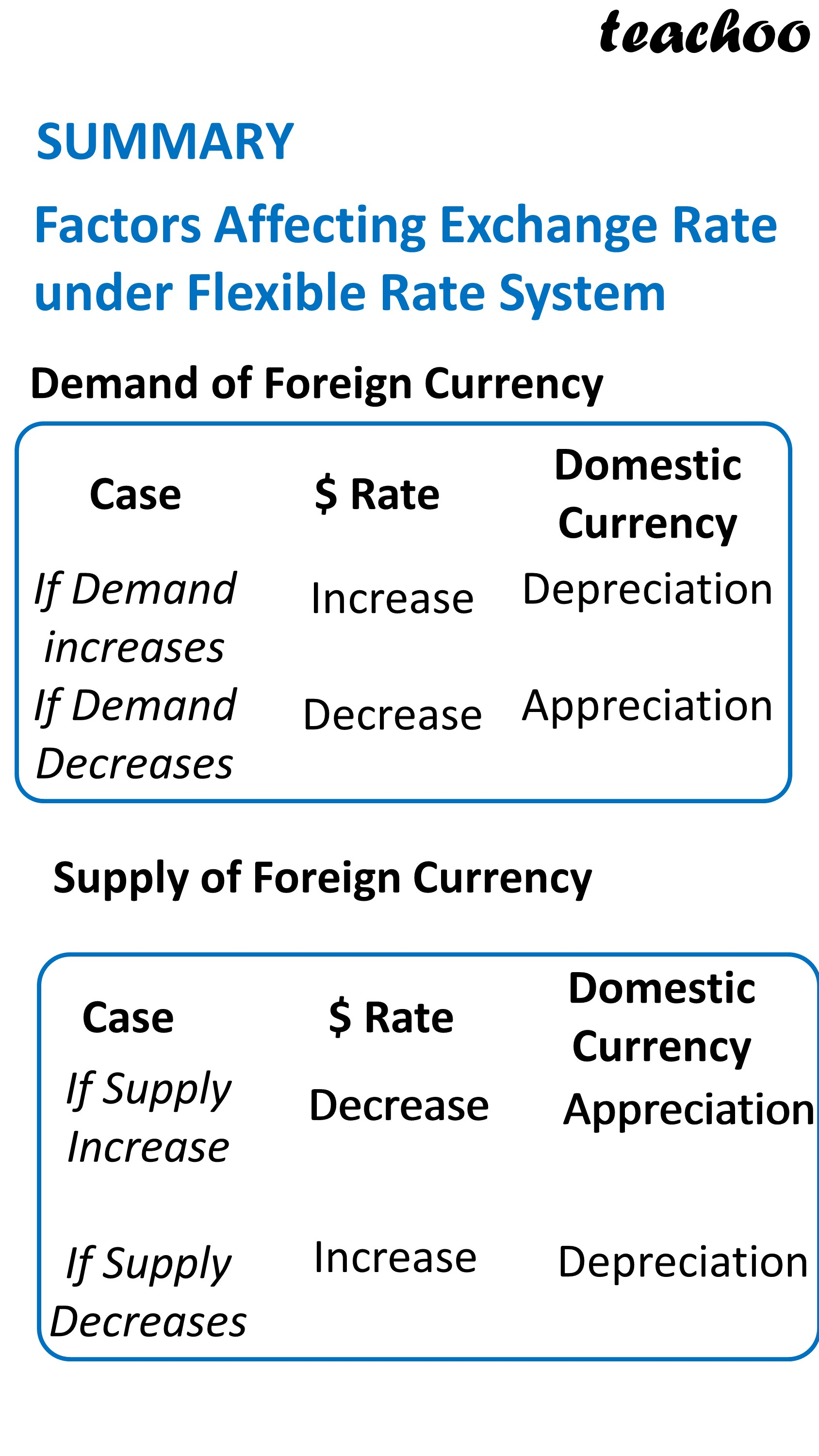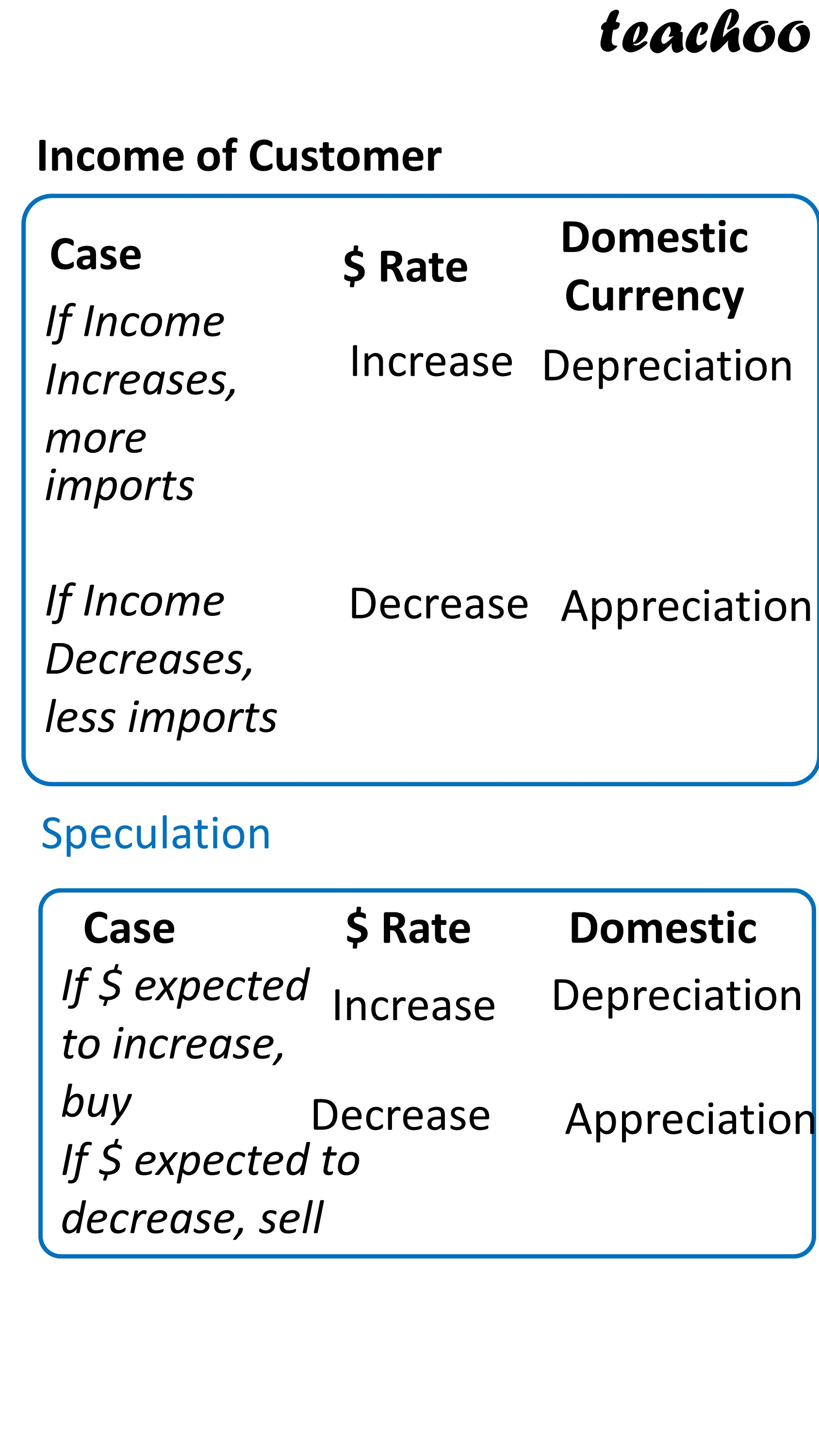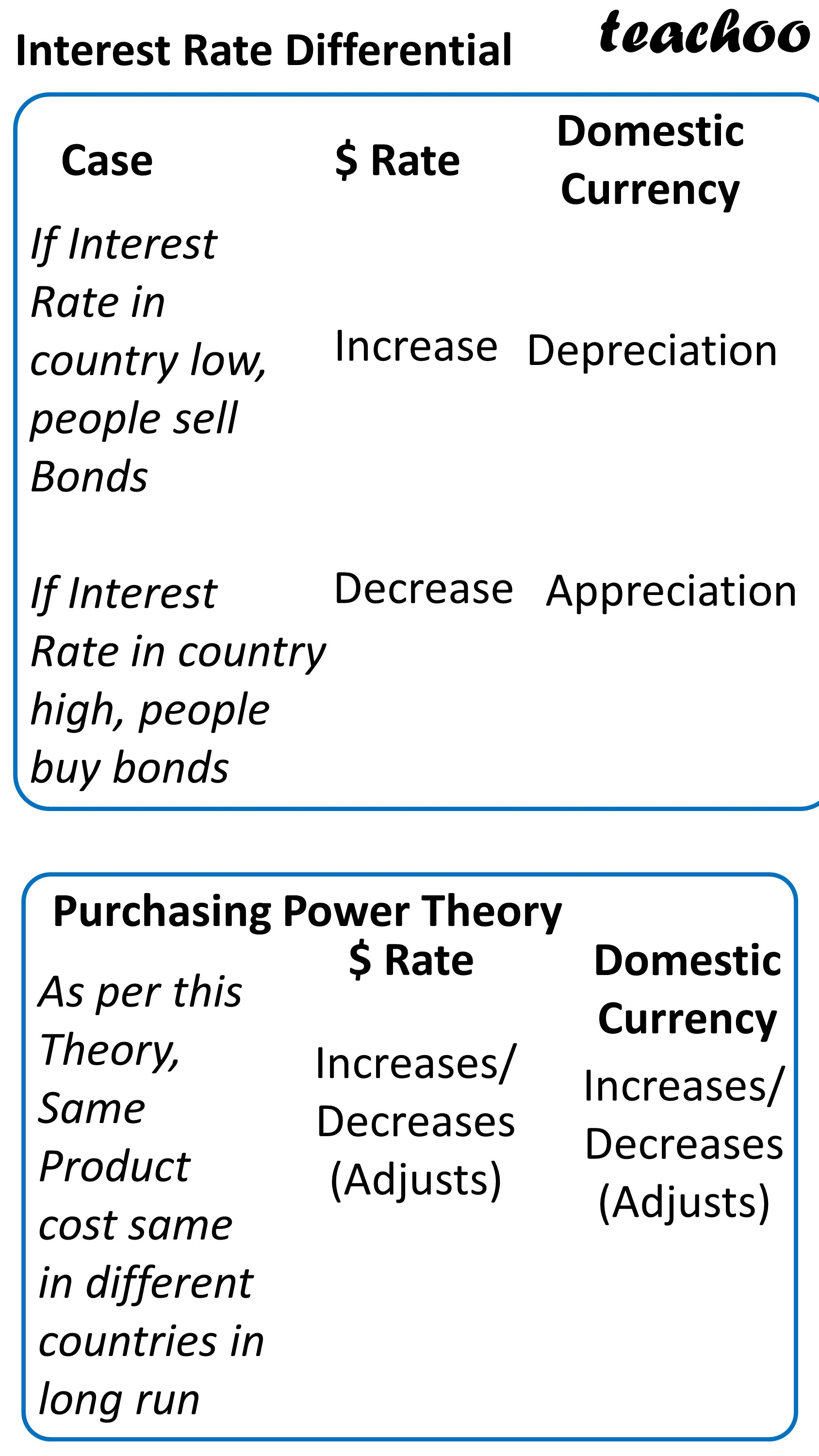Chapter 6 Part 1 - Foreign Exchange Rates

Economics Class 12
Macroeconomics

Summary of Flexible or Floating Exchange Rate System### NCERT Questions

#### Question 6

How is the exchange rate determined under a flexible exchange rate regime

### Other Books

#### Question 1

In the following questions, select the correct answers:

Flexible exchange rate system is also known as :

1. Pegged Exchange rate system
2. Dirty Floating
3. Floating exchange rate system
4. Both B and C

Learn in your speed, with individual attention - Teachoo Maths 1-on-1 Class

### Transcript

What is Flexible or Floating Exchange Rate Year 2020 2021 2022 Exchange Rate 70 75 80 In this case, Exchange Rate is determined by forces of Demand and Supply Demand of Foreign Exchange Supply of Foreign Exchange More the Exchange Rate Less the Foreign Exchange Demand (it is Inversely Proportional) More the Exchange Rate More the Foreign Exchange Supply (it is Directly Proportional) SUMMARY Factors Affecting Exchange Rate under Flexible Rate System Demand of Foreign Currency Case If Demand increases If Demand Decreases \$ Rate Increase Decrease Domestic Currency Depreciation Appreciation Supply of Foreign Currency Case If Supply Increase If Supply Decreases \$ Rate Decrease Increase Domestic Currency Appreciation Depreciation Income of Customer Case If Income Increases, more imports If Income Decreases, less imports \$ Rate Increase Decrease Appreciation If \$ expected to increase, buy If \$ expected to decrease, sell Increase Decrease Domestic Depreciation Appreciation Interest Rate Differential Case If Interest Rate in country low, people sell Bonds If Interest Rate in country high, people buy bonds \$ Rate Increase Decrease Domestic Currency Depreciation Appreciation Purchasing Power Theory As per this Theory, Same Product cost same in different countries in long run \$ Rate Increases/ Decreases (Adjusts) Domestic Currency Increases/ Decreases (Adjusts)